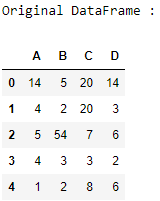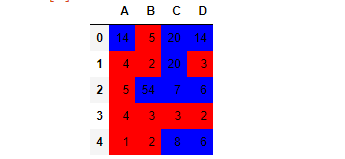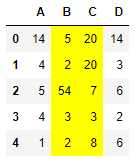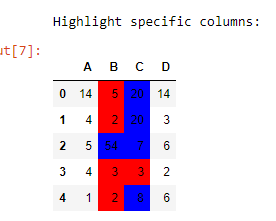# Highlight Pandas DataFrame’s specific columns using applymap()

Let us see how to highlight elements and specific columns of a Pandas DataFrame. We can do this using the `applymap()` function of the Styler class.

## Styler.applymap()

Syntax : Styler.applymap(self, func, subset = None, **kwargs)

Parameters :

• func : takes a scalar and returns a scalar.
• subset : valid indexer to limit data to before applying the function.
• **kwargs : dict pass along to func.

Returns : Styler

Let’s understand with examples:

First of all create a simple data frame:

 `# importing pandas as pd  ` `import` `pandas as pd  ` ` `  `# creating the dataframe   ` `df ``=` `pd.DataFrame({``"A"` `: [``14``, ``4``, ``5``, ``4``, ``1``],  ` `                   ``"B"` `: [``5``, ``2``, ``54``, ``3``, ``2``], ` `                   ``"C"` `: [``20``, ``20``, ``7``, ``3``, ``8``], ` `                   ``"D"` `: [``14``, ``3``, ``6``, ``2``, ``6``]})  ` ` `  `print``(``"Original DataFrame :"``) ` `display(df) `

Output :Example 1 : For every cell in the DataFrame, if the value is less than 6 then we will highlight the cell with red color, otherwise with blue color.

 `# function definition ` `def` `highlight_cols(s): ` `    ``color ``=` `'red'` `if` `s < ``6` `else` `'blue'` `    ``return` `'background-color: % s'` `%` `color ` ` `  `# highlighting the cells ` `display(df.style.applymap(highlight_cols)) `

Output :Example 2 : This time we will highlight only the cells in some specified columns.

 `# function definition ` `def` `highlight_cols(s): ` `    ``return` `'background-color: % s'` `%` `'yellow'` ` `  `# highlighting the cells ` `display(df.style.applymap(highlight_cols,  ` `                          ``subset ``=` `pd.IndexSlice[:, [``'B'``, ``'C'``]])) `

Output :Highlight specific columns with the help of Indexing:

## Python3

 `df.style.applymap(highlight_cols, subset ``=` `pd.IndexSlice[:, [``'B'``, ``'C'``]])`Attention geek! Strengthen your foundations with the Python Programming Foundation Course and learn the basics.

To begin with, your interview preparations Enhance your Data Structures concepts with the Python DS Course.

My Personal Notes arrow_drop_upCheck out this Author's contributed articles.

If you like GeeksforGeeks and would like to contribute, you can also write an article using contribute.geeksforgeeks.org or mail your article to contribute@geeksforgeeks.org. See your article appearing on the GeeksforGeeks main page and help other Geeks.

Please Improve this article if you find anything incorrect by clicking on the "Improve Article" button below.

Article Tags :

3

Please write to us at contribute@geeksforgeeks.org to report any issue with the above content.